# series definição, series significado | dicionário de inglês definição

Procurar também em: Site Notícias Enciclopédia Imagens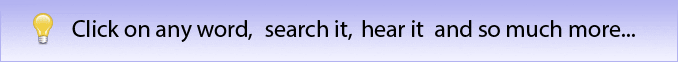## series

n   pl   , -ries
1    a group or connected succession of similar or related things, usually arranged in order
2    a set of radio or television programmes having the same characters and setting but different stories
3    a set of books having the same format, related content, etc., published by one firm
4    a set of stamps, coins, etc., issued at a particular time
5      (Maths)   the sum of a finite or infinite sequence of numbers or quantities
6      (Electronics)
a    a configuration of two or more components connected in a circuit so that the same current flows in turn through each of them (esp. in the phrase in series)
b    (as modifier)
a series circuit         Compare       parallel       10
7      (Rhetoric)   a succession of coordinate elements in a sentence
8      (Geology)   a stratigraphical unit that is a subdivision of a system and represents the rocks formed during an epoch
(C17: from Latin: a row, from serere to link)

actinide series
n   a series of 15 radioactive elements with increasing atomic numbers from actinium to lawrencium
actinium series
n   a radioactive series that starts with uranium-235 and ends with lead-207
electrochemical series
n      another name for       electromotive series
electromotive series
n     (Chem)   a series of the metals, together with hydrogen, ranged in the order of their electrode potentials
ethylene series
n     (Chem)   the homologous series of unsaturated hydrocarbons that contain one double bond and have the general formula, CnH2n; alkene series
Fourier series
n   an infinite trigonometric series of the form ½a0 + a1cos x + b1sin x + a2cos 2x + b2sin 2x + ..., where a0, a1, b1, a2, b2 ... are the Fourier coefficients. It is used, esp. in mathematics and physics, to represent or approximate any single-valued periodic function by assigning suitable values to the coefficients
(C19: named after Baron Jean Baptiste Joseph Fourier)
geometric series
n   a geometric progression written as a sum, as in 1 + 2 + 4 + 8
harmonic series
n
1      (Maths)   a series whose terms are in harmonic progression, as in 1 + ½ + 1/3 + ...
2      (Acoustics)   the series of tones with frequencies strictly related to one another and to the fundamental tone, as obtained by touching lightly the node points of a string while playing it. Its most important application is in the playing of brass instruments
lanthanide series
n   a class of 15 chemically related elements with atomic numbers from 57 (lanthanum) to 71 (lutetium)
Maclaurin's series
n     (Maths)   an infinite sum giving the value of a function f(x) in terms of the derivatives of the function evaluated at zero: f(x) = f(0) + (f′(0)x)/1! + (f″(0)x2)/2! + ...,   (Also called)    Maclaurin series
(C18: named after Colin Maclaurin (1698--1746), British mathematician who formulated it)
methane series
n      another name for the       alkane series

See
alkane
neptunium series
n   a radioactive series that starts with plutonium-241 and ends with bismuth-209. Neptunium-237 is the longest-lived member of the series. The series does not occur in nature
power series
n   a mathematical series whose terms contain ascending positive integral powers of a variable, such as a0 + a1x + a2x2 +...
n     (Physics)   a series of nuclides each of which undergoes radioactive decay into the next member of the series, ending with a stable element, usually lead
See       uranium series       neptunium series       thorium series       actinium series
series resonance
n   the resonance that results when circuit elements are connected with their inductance and capacitance in series, so that the impedance of the combination falls to a minimum at the resonant frequency
Compare       parallel resonance
series-wound
adj   (of a motor or generator) having the field and armature circuits connected in series
Compare       shunt-wound
Taylor's series
n     (Maths)   an infinite sum giving the value of a function f(z) in the neighbourhood of a point a in terms of the derivatives of the function evaluated at a. Under certain conditions, the series has the form f(z) = f(a) + [f′(a)(z -- a)]/1! + [f″(a)(z -- a)2]/2! + ...
(C18: named after B. Taylor)
thorium series
n   a radioactive series that starts with thorium--232 and ends with lead--208
time series
n     (Statistics)   a series of values of a variable taken in successive periods of time
uranium series
World Series   , World's Series
n     (Baseball)   (in the U.S.) a best-of-seven playoff for the world championship between the two winning teams in the major leagues at the end of the season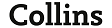Fibonacci sequence   , series
n   the infinite sequence of numbers, 0, 1, 1, 2, 3, 5, 8, etc., in which each member (Fibonacci number) is the sum of the previous two
(named after Leonardo Fibonacci)

actinide series
n   a series of 15 radioactive elements with increasing atomic numbers from actinium to lawrencium
actinium series
n   a radioactive series that starts with uranium-235 and ends with lead-207
electrochemical series
n      another name for       electromotive series
electromotive series
n     (Chem)   a series of the metals, together with hydrogen, ranged in the order of their electrode potentials
ethylene series
n     (Chem)   the homologous series of unsaturated hydrocarbons that contain one double bond and have the general formula, CnH2n; alkene series
Fourier series
n   an infinite trigonometric series of the form ½a0 + a1cos x + b1sin x + a2cos 2x + b2sin 2x + ..., where a0, a1, b1, a2, b2 ... are the Fourier coefficients. It is used, esp. in mathematics and physics, to represent or approximate any single-valued periodic function by assigning suitable values to the coefficients
(C19: named after Baron Jean Baptiste Joseph Fourier)
geometric series
n   a geometric progression written as a sum, as in 1 + 2 + 4 + 8
harmonic series
n
1      (Maths)   a series whose terms are in harmonic progression, as in 1 + ½ + 1/3 + ...
2      (Acoustics)   the series of tones with frequencies strictly related to one another and to the fundamental tone, as obtained by touching lightly the node points of a string while playing it. Its most important application is in the playing of brass instruments
lanthanide series
n   a class of 15 chemically related elements with atomic numbers from 57 (lanthanum) to 71 (lutetium)
Maclaurin's series
n     (Maths)   an infinite sum giving the value of a function f(x) in terms of the derivatives of the function evaluated at zero: f(x) = f(0) + (f′(0)x)/1! + (f″(0)x2)/2! + ...,   (Also called)    Maclaurin series
(C18: named after Colin Maclaurin (1698--1746), British mathematician who formulated it)
methane series
n      another name for the       alkane series

See
alkane
neptunium series
n   a radioactive series that starts with plutonium-241 and ends with bismuth-209. Neptunium-237 is the longest-lived member of the series. The series does not occur in nature
power series
n   a mathematical series whose terms contain ascending positive integral powers of a variable, such as a0 + a1x + a2x2 +...
n     (Physics)   a series of nuclides each of which undergoes radioactive decay into the next member of the series, ending with a stable element, usually lead
See       uranium series       neptunium series       thorium series       actinium series
series
n   pl   , -ries
1    a group or connected succession of similar or related things, usually arranged in order
2    a set of radio or television programmes having the same characters and setting but different stories
3    a set of books having the same format, related content, etc., published by one firm
4    a set of stamps, coins, etc., issued at a particular time
5      (Maths)   the sum of a finite or infinite sequence of numbers or quantities
6      (Electronics)
a    a configuration of two or more components connected in a circuit so that the same current flows in turn through each of them (esp. in the phrase in series)
b    (as modifier)
a series circuit         Compare       parallel       10
7      (Rhetoric)   a succession of coordinate elements in a sentence
8      (Geology)   a stratigraphical unit that is a subdivision of a system and represents the rocks formed during an epoch
(C17: from Latin: a row, from serere to link)
series resonance
n   the resonance that results when circuit elements are connected with their inductance and capacitance in series, so that the impedance of the combination falls to a minimum at the resonant frequency
Compare       parallel resonance
series-wound
adj   (of a motor or generator) having the field and armature circuits connected in series
Compare       shunt-wound
Taylor's series
n     (Maths)   an infinite sum giving the value of a function f(z) in the neighbourhood of a point a in terms of the derivatives of the function evaluated at a. Under certain conditions, the series has the form f(z) = f(a) + [f′(a)(z -- a)]/1! + [f″(a)(z -- a)2]/2! + ...
(C18: named after B. Taylor)
thorium series
n   a radioactive series that starts with thorium--232 and ends with lead--208
time series
n     (Statistics)   a series of values of a variable taken in successive periods of time
uranium series
World Series   , World's Series
n     (Baseball)   (in the U.S.) a best-of-seven playoff for the world championship between the two winning teams in the major leagues at the end of the seasontone row   , series
n     (Music)   a group of notes having a characteristic pattern or order that forms the basis of the musical material in a serial composition, esp. one consisting of the twelve notes of the chromatic scale,   (Also called)    note row      See also       serialism       twelve-tone

actinide series
n   a series of 15 radioactive elements with increasing atomic numbers from actinium to lawrencium
actinium series
n   a radioactive series that starts with uranium-235 and ends with lead-207
electrochemical series
n      another name for       electromotive series
electromotive series
n     (Chem)   a series of the metals, together with hydrogen, ranged in the order of their electrode potentials
ethylene series
n     (Chem)   the homologous series of unsaturated hydrocarbons that contain one double bond and have the general formula, CnH2n; alkene series
Fourier series
n   an infinite trigonometric series of the form ½a0 + a1cos x + b1sin x + a2cos 2x + b2sin 2x + ..., where a0, a1, b1, a2, b2 ... are the Fourier coefficients. It is used, esp. in mathematics and physics, to represent or approximate any single-valued periodic function by assigning suitable values to the coefficients
(C19: named after Baron Jean Baptiste Joseph Fourier)
geometric series
n   a geometric progression written as a sum, as in 1 + 2 + 4 + 8
harmonic series
n
1      (Maths)   a series whose terms are in harmonic progression, as in 1 + ½ + 1/3 + ...
2      (Acoustics)   the series of tones with frequencies strictly related to one another and to the fundamental tone, as obtained by touching lightly the node points of a string while playing it. Its most important application is in the playing of brass instruments
lanthanide series
n   a class of 15 chemically related elements with atomic numbers from 57 (lanthanum) to 71 (lutetium)
Maclaurin's series
n     (Maths)   an infinite sum giving the value of a function f(x) in terms of the derivatives of the function evaluated at zero: f(x) = f(0) + (f′(0)x)/1! + (f″(0)x2)/2! + ...,   (Also called)    Maclaurin series
(C18: named after Colin Maclaurin (1698--1746), British mathematician who formulated it)
methane series
n      another name for the       alkane series

See
alkane
neptunium series
n   a radioactive series that starts with plutonium-241 and ends with bismuth-209. Neptunium-237 is the longest-lived member of the series. The series does not occur in nature
power series
n   a mathematical series whose terms contain ascending positive integral powers of a variable, such as a0 + a1x + a2x2 +...
n     (Physics)   a series of nuclides each of which undergoes radioactive decay into the next member of the series, ending with a stable element, usually lead
See       uranium series       neptunium series       thorium series       actinium series
series
n   pl   , -ries
1    a group or connected succession of similar or related things, usually arranged in order
2    a set of radio or television programmes having the same characters and setting but different stories
3    a set of books having the same format, related content, etc., published by one firm
4    a set of stamps, coins, etc., issued at a particular time
5      (Maths)   the sum of a finite or infinite sequence of numbers or quantities
6      (Electronics)
a    a configuration of two or more components connected in a circuit so that the same current flows in turn through each of them (esp. in the phrase in series)
b    (as modifier)
a series circuit         Compare       parallel       10
7      (Rhetoric)   a succession of coordinate elements in a sentence
8      (Geology)   a stratigraphical unit that is a subdivision of a system and represents the rocks formed during an epoch
(C17: from Latin: a row, from serere to link)
series resonance
n   the resonance that results when circuit elements are connected with their inductance and capacitance in series, so that the impedance of the combination falls to a minimum at the resonant frequency
Compare       parallel resonance
series-wound
adj   (of a motor or generator) having the field and armature circuits connected in series
Compare       shunt-wound
Taylor's series
n     (Maths)   an infinite sum giving the value of a function f(z) in the neighbourhood of a point a in terms of the derivatives of the function evaluated at a. Under certain conditions, the series has the form f(z) = f(a) + [f′(a)(z -- a)]/1! + [f″(a)(z -- a)2]/2! + ...
(C18: named after B. Taylor)
thorium series
n   a radioactive series that starts with thorium--232 and ends with lead--208
time series
n     (Statistics)   a series of values of a variable taken in successive periods of time
uranium series
World Series   , World's Series
n     (Baseball)   (in the U.S.) a best-of-seven playoff for the world championship between the two winning teams in the major leagues at the end of the season## series

arrangement, chain, course, line, order, progression, run, sequence, set, string, succession, train

Consulte também:
Dicionário Colaborativo     Inglês Definição
 n. a series of tweets on the same topic published in a short period [Tech.]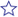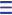binge-watch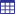v. watch multiple episodes of a television program such as a series in a row On the pattern of 'binge-eat' or 'binge-drink', the term 'binge' meaning an excessive indulgence in something.Ex: As I became bored, I decided to binge-watch the entire season of my favorite series exp. series of functions performed by an electronic system independently of (not connected to) the cyberspace.n. watch a great number of episodes from the same television series in a row portmanteau word: binge (excessive consumption of something) + watchingn. series of concentric, expanding circles caused by ripples in water from a central pointexp. (about a movie or TV series) reach a point when, due to a unauthentic scene, it loses the appreciation of the public made popular by "Indiana Jones" whose hero survives an explosion by hiding in a fridgePara acrescentar entradas à sua lista de vocabulário, junte-se à nossa comunidade. É fácil e rápido: